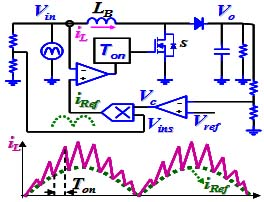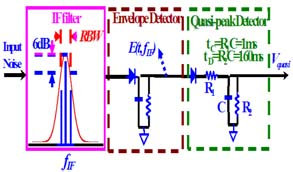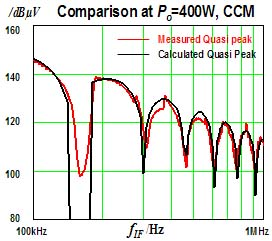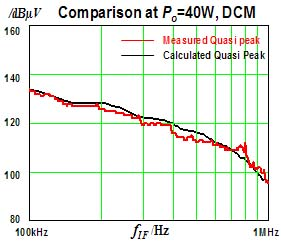LIBRARY

# DM EMI Noise Prediction in Constant On-time PFC

Year: 2010Fig. 1. Constant On-time Control Scheme in CCM.
Constant on-time control shown as in Fig. 1, which is widely used in DC-DC converters, could be a potential candidate in PFC to boost light load efficiency.

A major concern for constant on-time PFC is the conducted EMI noise. Most of the previous research mainly focus on the EMI noise analysis of constant-frequency PFC. EMI noise characteristics of constant on-time PFC, a variable-frequency PFC, are unclear and have not been carefully investigated.

In this paper, the principle of the quasi-peak EMI noise measurement process is investigated and simplified as shown in Fig. 2 and a mathematical model is developed to predict the quasi-peak EMI noise.

A 400W digitally controlled constant on-time PFC is built to verify the mathematical model and the quasi-peak DM noise prediction. The test condition is, input RMS voltage Vrms=110V, Vo=400V, LB=270µH and Ton=7µs for Po=400W (CCM) and Po=40W (DCM). The comparison results are shown in Fig. 3 and Fig. 4, respectively. We can see that the experimental results prove the accuracy of the prediction.Fig. 2. Simplified Block Diagram of Quasi-peak Noise Detection.Fig. 3. Quasi-peak Noise Prediction for Constant On-time PFC in CCM.Fig. 4. Quasi-peak Noise Prediction for Constant On-time PFC in DCM.